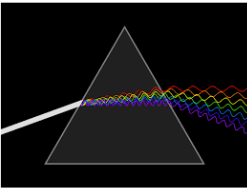# Prism theory and Dispersion of light MCQs for NEET

A prism has three rectangular surfaces and two triangular faces inclined at an angle. The angle between the two refracting surfaces is called the angle of the prism. The angle between the incident ray and the emergent ray is called the angle of deviation of the prism. Dispersion is defined as the separation of white light into different colours when the light is passed through the prism. The amount of scattering depends on the wavelength of light.Q1: The critical angle between an equilateral prism and air is 45. If the incident ray is perpendicular to the refracting surface, then

1. It is reflected totally from the second surface and emerges perpendicular from the third surface.
2. It gets reflected from second and third surfaces and emerges from the first surface
3. It keeps reflecting from all the three sides of the prism and never emerges out
4. After deviation, it gets refracted from the second surface

Answer: (a) It is reflected totally from the second surface and emerges perpendicular from the third surface.

Q2: Which ray is least deviated by a prism?

1. Violet ray
2. Green ray
3. Red ray
4. Yellow ray

Q3: A prism (μ=1.5) has a refracting angle of 300. The deviation of a monochromatic ray incident normally on its one surface will be

1. 180 36’
2. 200 30’
3. 180
4. 190 30’

Q4: The angle of the prism is equal to the angle of minimum deviation for a prism of refractive index 1.5. What is the value of the angle of the prism?

1. 410
2. 820
3. 620
4. 310

Q5: The dispersive power of prism depends upon

1. The shape of the prism
2. The material of the prism
3. The angle of the prism
4. Height of the prism

Answer: (b) The material of the prism

Q6: When white light passes through the achromatic combination of prism, then what is observed

1. Dispersion
2. Deviation
3. Dispersion and deviation
4. None of the above

Q7: For a wavelength l the dispersion of the medium is D, then the dispersion for the wavelength 2l will be

1. D/8
2. D/2
3. 2D
4. D

Q8: The refractive angle of a prism for a monochromatic light is 600 and refractive index is √2. For minimum deviation, the angle of incidence will be

1. 600
2. 450
3. 300
4. 750

Q9: The dispersive power will be maximum for

1. Flint glass
2. Crown glass
3. Mixture of glass
4. None of these

Q10: When white light is passed through a hollow prism then there is

1. no dispersion and no deviation
2. Only dispersion
3. Only deviation
4. Both dispersion and deviation

Answer: (a) no dispersion and no deviation